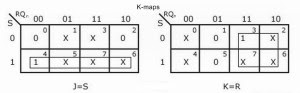# Conversion of JK flip flop to SR flip flop, T and D flip flop

Hello friends, in this article, we will learn Conversion of JK flip flop to SR, T, and D flip flop. We will cover, introduction, Conversion tables, Logic diagrams, and K-maps for the same.

## Conversion of JK flip flop

### Introduction:

We have discussed “how to convert SR flip flop into JK and D type of flip flop” in the last article. Now we are going to convert JK flip flop into different types of flip-flops.

As explained in the last article, if we want to convert one type of flip flop into another type of flip flop, first we have to design a combinational circuit and after that connect it to the inputs of the actual flip flop. So that the outputs of the combinational circuit will be the inputs of the actual flip flop and then it will produce the same output as that of the required flip flop.

We can convert JK flip flop into SR, T, and D type of flip-flops.

### 1)      Conversion of JK flip flop to SR flip flop:

In case of converting JK flip flop into SR flip flop, external inputs (inputs of a combinational circuit) are S and R, while J and K are the inputs of the actual flip flop.

So we have to get values of J and K in terms of S, R, and Qn. Thus we prepare a conversion table S, R, Qn, Qn+1, J and K.

The external inputs S and R and the output Qn can make 8 combinations. For each combination find the corresponding Qn+1.

In the SR flip flop, the combination S=1 and R=1 is not permitted. So, the corresponding output is invalid and, therefore the corresponding J and K are don’t cares.

Complete the table by writing the values of J and K required getting each Qn+1 from the corresponding Qn.

The conversion table, K-maps and Logic diagram for the conversion of JK flip flop to SR flip flop is shown below:

##K-Maps

### 2)      Conversion of JK flip flop to T flip flop:

For the conversion of JK flip flop to T type of flip flop, T will be the external input (input of combinational circuit) and the output of this combinational circuit is connected to the inputs of actual flip flop (J and K).

Then we prepare a conversion table and using this table express J and K in terms of T and Qn.

The conversion table, K-Maps and logic diagram for the conversion of JK flip flop to T type of flip flop is shown below:

### 3)      Conversion of JK flip flop to D flip flop:

In case of converting JK flip flop into D flip flop, D is the external input of the combinational circuit, whereas J and K are the inputs of the actual flip flop.

D and Qn make four combinations. So, prepare a conversion table and using this table express J and K in terms of D and Qn.

The conversion table, K-map and logic diagram for the conversion of JK flip flop to D flip flop is shown below: